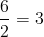Sunday , 23 January 2022

Basic Math Symbols

Basic Math Symbols – List of all basic mathematical symbols and signs – meaning and examples.

Symbol Symbol Name Meaning / definition Example
= equals sign equality 5 = 2 + 3
> strict inequality greater than 5 > 4
< strict inequality less than 4 < 5
inequality greater than or equal to 5 ≥ 4
inequality less than or equal to 4 ≤ 5
( ) parentheses calculate expression inside first 2 × (3+5) = 16
[ ] brackets calculate expression inside first [(1+2)*(1+5)] = 18
+ plus sign addition 1 + 1 = 2
minus sign subtraction 2 − 1 = 1
± plus – minus both plus and minus operations 3 ± 5 = 8 and -2
minus – plus both minus and plus operations 3 ∓ 5 = -2 and 8
* asterisk multiplication 2 * 3 = 6
× times sign multiplication 2 × 3 = 6
multiplication dot multiplication 2 ∙ 3 = 6
÷ division sign / obelus division 6 ÷ 2 = 3
/ division slash division 6 / 2 = 3
horizontal line division / fractionmod modulo remainder calculation 7 mod 2 = 1
. period decimal point, decimal separator 2.56 = 2+56/100
ab power exponent 23 = 8
a^b caret exponent 2 ^ 3 = 8
a square root a · a  = a √9 = ±3
3a cube root 33√a  · 3√a  =a 3√8 = 2
4a fourth root 4a · 4√a  · 4√a  · 4√a  = a 4√16 = ±2
na n-th root (radical) for n=3, n√8 = 2
% percent 1% = 1/100 10% × 30 = 3
per-mille 1‰ = 1/1000 = 0.1% 10‰ × 30 = 0.3
ppm per-million 1ppm = 1/1000000 10ppm × 30 = 0.0003
ppb per-billion 1ppb = 1/1000000000 10ppb × 30 = 3×10-7
ppt per-trillion 1ppt = 10-12 10ppt × 30 = 3×10-10

Scorpio Weekly Horoscope January 2022

Scorpio Weekly Horoscope (October 23 – November 21) Scorpio, with the symbol of ‘The Scorpion’, …